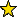# RelativeCanonicalResolution -- construction of relative canonical resolutions and Eagon-Northcott type complexes

## Description

This package provides functions that construct g-nodal canonical curves with a degree k line bundle that lie on a normalized scroll. It furthermore contains functions that compute the relative canonical resolution. The construction of such canonical curves is based on the Macaulay2 package kGonalNodalCurves. This package can be seen as an upgrade to the kGonalNodalCurves package.

We also provide functions to compute (possibly non-minimal) free resolutions of such curves by an iterated mapping cone construction, as described in Schreyer's article Syzygies of Canonical Curves and Special Linear Series.

## Construction of relative canonical resolutions

• canCurveWithFixedScroll -- Computes a g-nodal canonical curve with a degree k line bundle on a normalized scroll
• curveOnScroll -- Computes the ideal of a canonical curve on a normalized scroll in terms of generators of the scroll
• resCurveOnScroll -- Computes the relative canonical resolution

## Iterated mapping cones and Eagon-Nortcott type complexes

• eagonNorthcottType -- Computes the Eagon-Northcott type resolution
• liftMatrixToEN -- Lifts a matrix between bundles on the scroll to the associated Eagon-Northcott type complexes
• iteratedCone -- Computes a (possibly non-minimal) resolution of C in P^{g-1} starting from the relative canonical resolution of C in P(E)

## CertificationVersion 1.0 of this package was accepted for publication in volume 11 of The Journal of Software for Algebra and Geometry on 28 August 2020, in the article The relative canonical resolution: Macaulay2-package, experiments and conjectures. That version can be obtained from the journal or from the Macaulay2 source code repository.

## Version

This documentation describes version 1.0 of RelativeCanonicalResolution.

## Source code

The source code from which this documentation is derived is in the file RelativeCanonicalResolution.m2.

## Exports

• Functions and commands
• balancedPartition -- Computes balanced partition of n of length d
• canCurveWithFixedScroll -- Computes a g-nodal canonical curve with a degree k line bundle on a normalized scroll
• canonicalMultipliers -- Computes the canonical multipliers of a rational curves with nodes
• coxDegrees -- Computes the degree of a polynomial in the Cox ring corresponding to a section of a bundle on the scroll
• curveOnScroll -- Computes the ideal of a canonical curve on a normalized scroll in terms of generators of the scroll
• eagonNorthcottType -- Computes the Eagon-Northcott type resolution
• iteratedCone -- Computes a (possibly non-minimal) resolution of C in P^{g-1} starting from the relative canonical resolution of C in P(E)
• liftMatrixToEN -- Lifts a matrix between bundles on the scroll to the associated Eagon-Northcott type complexes
• lineBundleFromPointsAndMultipliers -- Computes basis of a line bundle from the 2g points P_i, Q_i and the multipliers
• resCurveOnScroll -- Computes the relative canonical resolution
• rkSyzModules -- Computes the rank of the i-th module in the relative canonical resolution
• scrollDegrees -- Computes the degree of a section of a bundle on the scroll ring corresponding to a polynomial in the Cox ring
• Methods
• "balancedPartition(ZZ,ZZ)" -- see balancedPartition -- Computes balanced partition of n of length d
• "canCurveWithFixedScroll(ZZ,ZZ,ZZ)" -- see canCurveWithFixedScroll -- Computes a g-nodal canonical curve with a degree k line bundle on a normalized scroll
• "canonicalMultipliers(Matrix,Matrix)" -- see canonicalMultipliers -- Computes the canonical multipliers of a rational curves with nodes
• "coxDegrees(List,List)" -- see coxDegrees -- Computes the degree of a polynomial in the Cox ring corresponding to a section of a bundle on the scroll
• "curveOnScroll(Ideal,ZZ,ZZ)" -- see curveOnScroll -- Computes the ideal of a canonical curve on a normalized scroll in terms of generators of the scroll
• "eagonNorthcottType(Matrix,ZZ)" -- see eagonNorthcottType -- Computes the Eagon-Northcott type resolution
• "iteratedCone(ChainComplex,List)" -- see iteratedCone -- Computes a (possibly non-minimal) resolution of C in P^{g-1} starting from the relative canonical resolution of C in P(E)
• "liftMatrixToEN(Matrix,List)" -- see liftMatrixToEN -- Lifts a matrix between bundles on the scroll to the associated Eagon-Northcott type complexes
• "lineBundleFromPointsAndMultipliers(List,Matrix,Matrix,ZZ)" -- see lineBundleFromPointsAndMultipliers -- Computes basis of a line bundle from the 2g points P_i, Q_i and the multipliers
• "resCurveOnScroll(Ideal,ZZ)" -- see resCurveOnScroll -- Computes the relative canonical resolution
• "resCurveOnScroll(Ideal,ZZ,ZZ)" -- see resCurveOnScroll -- Computes the relative canonical resolution
• "rkSyzModules(ZZ,ZZ)" -- see rkSyzModules -- Computes the rank of the i-th module in the relative canonical resolution
• "scrollDegrees(List,List)" -- see scrollDegrees -- Computes the degree of a section of a bundle on the scroll ring corresponding to a polynomial in the Cox ring

## For the programmer

The object RelativeCanonicalResolution is .